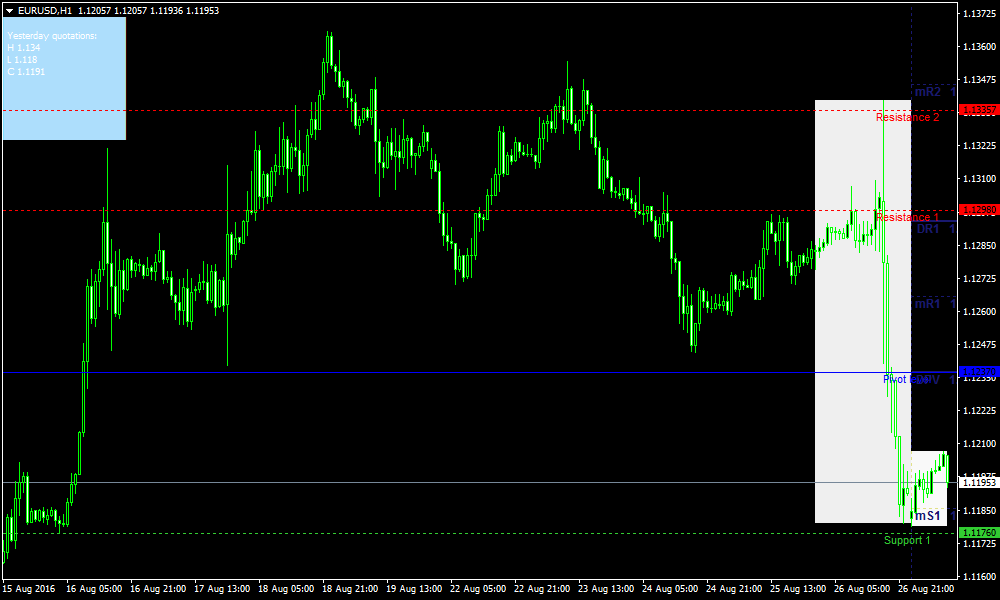### Trading Forex Pivot Points StrategyThis system uses a 15 min, 30min and 1 hour. This is applicable to all currency pairs.

## Forex Indicators:

• Pivot Points levels
• Fibonacci Support and Resistance

### SCENARIOS:

The price is below the Pivot but above S1.
• Buy above the pivot, (if price reaches above pivot)
• Sell below S1(if the prcies goes below S1).
The price is above Pivot but below R1.
• Buy above R1 (if the price reaches above R1).
• Sell below Pivot(if the price goes below Pivot).
The price is very near to pivot (+/- 0.02%)
• Buy above R1 (if the price reaches above R1).
• Sell below S1(if the price reaches below S1).
The price is between R1 and R2.
• Buy above R2 (if the price reaches above R2).
• Sell below Pivot(if the price reaches below Pivot). Take note, do not sell below R1. You must sell below Pivot.
The price is between S1 and S2.
• Buy above Pivot (if the price reaches above Pivot).
• Sell below S2(if the price reaches below S2). Take note, do not buy above S1, buy only above pivot.
The price is between S2 and S3.
• Same rule applies as rule 5. Buy above pivot sell below S3.
The price is between R2 and R3.
• Same rule applies as rule 4. Buy above R3 sell below Pivot.

### How to install Trading Forex Pivot Points Strategy?

• Copy mq4 and ex4 files to your Metatrader Directory / experts / indicators /
• Copy tpl file (Template) to your Metatrader Directory / templates /
• Select Chart and Timeframe where you want to test your forex strategy